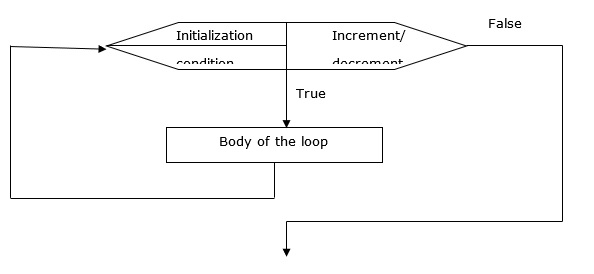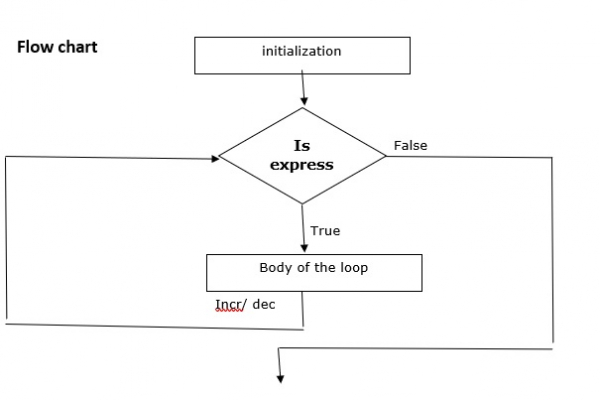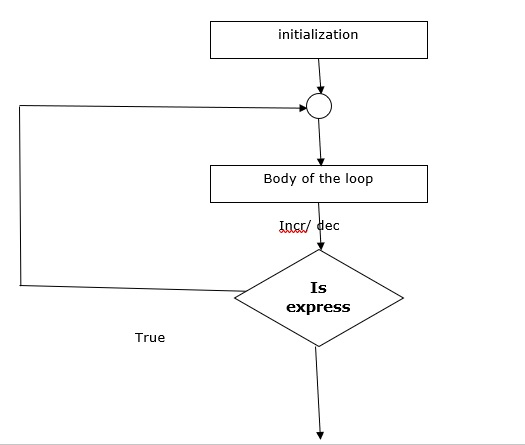# What are the loop control statements in C language? Explain with flow chart and program

Loop control statements are used to repeat set of statements. They are as follows −

• for loop
• while loop
• do-while loop

## for loop

The syntax is as follows −

for (initialization ; condition ; increment / decrement){
body of the loop
}

### Flow chart

The flow chart for loop is as follows −• Initialization is usually an assignment statement that is used to set the loop control variable.

• The condition is a relational expression that determines when the loop will exit.

• The increment/decrement part defines how the loop control variable will change each time loop is repeated.

• Loop continues to execute as long as the condition is true.

• Once the condition is false, program continues with the next statement after for loop.

## Example

Following is the C program for loop control statement −

Live Demo

#include<stdio.h>
main( ){
int k;
for (k = 1; k<=5; k++){
printf ("%d",k);
}
}

## Output

When the above program is executed, it produces the following result −

1 2 3 4 5

## while loop

The syntax is as follows −

while (condition){
body of the loop
}

### Flow chart

The flow chart for while loop is as follows −• Initialization is done before the loop.
• Loop continues as long as the condition is true.
• Increment and decrement part is done within the loop.

## Example

Following is the C program for while loop control statement −

Live Demo

#include<stdio.h>
main( ){
int k;
k = 1;
while (k<=5){
printf ("%d",k);
k++;
}
}

## Output

When the above program is executed, it produces the following result −

1 2 3 4 5

## do-while loop

The syntax is as follows −

Initialization
do{
body of the loop
inc/ dec
} while (condition);

### Flow chart

The flow chart for do-while loop is as follows −## Example

Following is the C program for do-while loop control statement −

Live Demo

#include<stdio.h>
main( ){
int k;
k = 1;
do{
printf ("%d",k);
k++;
}
while (k <= 5);
}

## Output

When the above program is executed, it produces the following result −

1 2 3 4 5